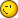# Gram-smith Process

2 replies to this topic

### #1Fabri

Fabri

Newbie

•• Members
•• 21 posts

• Calculators:
Casio fx 6300G

Posted 27 May 2006 - 12:02 AM

Hi my question is about Gram-Smith process.
This process could to have a ortogonal dimension of vector, family of vectors, i hope anyone knows this process, in classpad i cant made a program to solve that.
My problem is:
Its possible to store a matrix in a variable?
When i doing a thing similiar to this if v1=(a,b,c,...,n),v2=(a1,b1,c1,...,n1),v3=(a2,b2,c2,...,n2),vn=(ai,bi,ci,...,ni) VECTORS

Gram-Smith process is:
(<v2,w1> and others are dot product of vectors)
||wn|| is norm of vector

w1=v1 //main vector

w2= v2- ((<v2,w1>)/(||w1||?))*w1 //second vector ortogonal to w1//

w3= v3- ((<v3,w1>)/(||w1||?))*w1- ((<v3,w2>)/(||w2||?))*w2 //second vector ortogonal to w1//

wn= vn- ((<vn,v1>)/(||v1||?))*v1- ......((<v3,wn-1>)/(||wn-1||?))*wn-1 //n vector ortogonal to w1,w2,wn-1//

its possible to store w1 vector, w2 vector, wn vector, whit no overwrite a K variable (k its a ny variable)
i did do whit k={} but this notation doesnt support matrix stord in a variable and viceverza when i doing whit matrix and in that sotore a list

how does solve this problem???

### #2PAP

PAP

Casio Overlord

•• Members
•• 681 posts
• Gender:Male
• Location:Somewhere in Europe.
• Interests:Computer Algebra, Numerical Analysis.

• Calculators:
ClassPad 300 (plus an old Casio model, with only a few Kb ram).

Posted 27 May 2006 - 12:09 AM

The Gram-Schmidt method can be implemented in CPLua rather easily. I'm planning to a function for this in LuaNumAn, but it has a rather low priority, since the "todo" list is always full of more challenging methods. However, if you know CPLua, you can write your own implementation easily: you need to define a function implementing the inner product <a,b>, and a recursive function for the main Gram-Schmidt process.

### #3Daniel (CZE)

Daniel (CZE)

•• Members
•• 74 posts
• Location:Milevsko - Czech Republic
• Interests:Support for Pocket Viewer and ClassPad for Czech Republic.

• Calculators:
ClassPad 300, TI-92 Plus, FX-6300G, CFX-9850GB Plus

Posted 29 May 2006 - 01:10 PM

Hi my question is about Gram-Smith process.
This process could to have a ortogonal dimension of vector, family of vectors, i hope anyone knows this process, in classpad i cant made a program to solve that.
My problem is:
Its possible to store a matrix in a variable?
When i doing a thing similiar to this if v1=(a,b,c,...,n),v2=(a1,b1,c1,...,n1),v3=(a2,b2,c2,...,n2),vn=(ai,bi,ci,...,ni) VECTORS

Gram-Smith process is:
(<v2,w1> and others are dot product of vectors)
||wn|| is norm of vector

w1=v1 //main vector

w2= v2- ((<v2,w1>)/(||w1||?))*w1 //second vector ortogonal to w1//

w3= v3- ((<v3,w1>)/(||w1||?))*w1- ((<v3,w2>)/(||w2||?))*w2 //second vector ortogonal to w1//

wn= vn- ((<vn,v1>)/(||v1||?))*v1- ......((<v3,wn-1>)/(||wn-1||?))*wn-1 //n vector ortogonal to w1,w2,wn-1//

its possible to store w1 vector, w2 vector, wn vector, whit no overwrite a K variable (k its a ny variable)
i did do whit k={} but this notation doesnt support matrix stord in a variable and viceverza when i doing whit matrix and in that sotore a list

how does solve this problem???

I don't understand what do you exactly mean.

Storing vector in a variable is easy. Just use matrix notation for this. [1, 2, 3, 4]=>v1 where "=>" is store command.

If you want to create your variables in a program you can use # command. I show it in example in Main application.
```DelVar v1
done
"v1"=>varName
"v1"
[1,2,3,4]=>#varName
[1, 2, 3, 4]
v1
[1, 2, 3, 4]```

When using StrJoin function you can create vars in cycle with increasing number in name.

If I didn't write what you wanted then I'm sorry.PS: Sorry for my english#### 0 user(s) are reading this topic

0 members, 0 guests, 0 anonymous users